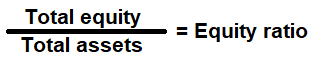# Equity Ratio Calculator

Key in the Total Equity and Total Assets Values from your Balance Sheet to the respective fields given below and then click Calculate to get the desired result.

# Equity Ratio Calculator - Glossary:

Equity Ratio: It shows the proportion of a company's assets that is financed by equity. It is also called "Equity multiplier".

### Formula:### How to use this equation?

This is a balance sheet component; the values are commonly stated against Total Equity and Total Assets. To use this ratio, divide the total equity by total assets.

Total Shareholder's Equity (Equity):
Total equity is the is the value left in the company after subtracting total liabilities from total assets.

Total Assets:
Total assets are the sum of all current and noncurrent assets that a company owns.

Example:
Equity ratio for a company with a total equity of \$150,000 and total assets of \$200,000 is 0.75:1. It means that, 75% of its assets are financed by equity and the remaining 25% is financed by debt.